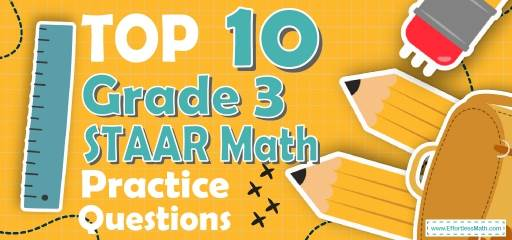# Top 10 3rd Grade STAAR Math Practice QuestionsPreparing 3rd-grade students for the STAAR Math test? The best way to prepare your students for the STAAR Math test is to work through as many STAAR Math practice questions as possible. Here are the top 10 3rd Grade STAAR Math practice questions to help your students review the most important 3rd Grade STAAR Math concepts. These 3rd Grade STAAR Math practice questions are designed to cover mathematics concepts and topics that are found on the actual test. The questions have been fully updated to reflect the latest 2022 STAAR guidelines. Answers and full explanations are provided at the end of the post.

## 3rd Grade STAAR Math Practice Questions

1- There are 6 numbers in the box below. Which of the following list shows only odd numbers from the numbers in the box?

A. $$15, 29, 42$$

B. $$15, 29, 83$$

C. $$15, 30, 42$$

D. $$42, 18, 30$$

2- Mia’s goal is to save $140 to purchase her favorite bike. In January, she saved$36.
In February, she saved $28. How much money does Mia need to save in March to be able to purchase her favorite bike? A.$28

B. $30 C.$52

D. $76 3- Michelle has 72 old books. She plans to send all of them to the library in their area. If she puts the books in boxes that can hold 9 books, which of the following equations can be used to find the number of boxes she will use? A. $$72 + 9 =$$ ______ B. $$72 × 9 =$$ ______ C. $$72 – 9 =$$ ______ D. $$72 ÷ 9 =$$ ______ 4- Eva had 983 cards. Then, she gave 349 of the cards to her friend Alice. After that, Eva lost 260 cards. Which equation can be used to find the number of cards Eve has now? A. $$983 – 349 + 260 =$$ _____ B. $$983 – 349 –260 =$$ _____ C. $$983 +349 +260 =$$ _____ D. $$983 +349 –260 =$$ _____ 5- The length of the following rectangle is 7 centimeters and its width is 3 centimeters. What is the area of the rectangle? A. $$10 cm^2$$ B. $$20 cm^2$$ C. $$14 cm^2$$ D. $$21 cm^2$$ 6- Which number is made up of 4 hundred, 8 tens, and 4 ones? A. 4084 B. 484 C. 448 D. 844 7- Look at the spinner above. On which color is the spinner most likely to land? A. RED B. GREEN C. YELLOW D. NONE 8- A group of third-grade students recorded the following distances that they jumped. A. 23 B. 24 C. 32 D. 34 9- Emma flew 2,448 miles from Los Angeles to New York City. What is the number of miles Emma flew rounded to the nearest thousand? A. 2000 B. 2400 C. 2500 D. 3000 10- A number sentence such as $$31 + Z = 98$$can be called an equation. If this equation is true, then which of the following equations is not true? A. $$98 – 31 = Z$$ B. $$98 – Z= 31$$ C. $$Z– 31 = 98$$ D. $$Z+31 = 98$$ ## Best 3rd Grade STAAR Math Prep Resource for 2022 ## Answers: 1- B An easy way to tell whether a large number is odd or even is to look at its final digit. If the number ends with an odd digit $$(1, 3, 5, 7, or 9)$$, then it’s odd. On the other hand, if the number ends with an even digit or $$0 (0, 2, 4, 6 or 8)$$, then it is even. 15, 29, and 83 ends with odd digits, so they are odd numbers. 2- D She saves$36 and $28 so she has$64.
$$140 – 64 = 76$$ she needs to save.

3- D
Michelle divides 72 books into 9 boxes therefore $$72 ÷ 9$$ formula is correct.

4- B
Eva gave 349 of her 983 cards to her friend so her cards are $$983-349$$, then she lost 260 cards so now she has $$983-349-260=374$$ cards

5- D
Use are formula of rectangle:
$$Area= length × width$$
Area $$=3cm×7cm =21cm^2$$

6- B
Add 4hundred and 8 tens and 4ones
So we have :$$400 + 80 + 4 = 484$$

7- A
The chances of landing on red are 3 in 6
The chances of landing on yellow are 1 in 6
The chances of landing on green are 2 in 6
The chances of landing on red are more than other colors.

8- C
$$32$$ inches distance most occur in this table.

9- A

10- C
All these equations are true:
$$98 – 31 = Z$$
$$98 – Z = 31$$
$$Z + 31 = 98$$

## The Best Books to Ace the 3rd Grade STAAR Math Test

### What people say about "Top 10 3rd Grade STAAR Math Practice Questions - Effortless Math: We Help Students Learn to LOVE Mathematics"?

No one replied yet.

X
30% OFF

Limited time only!

Save Over 30%

SAVE $5 It was$16.99 now it is \$11.99